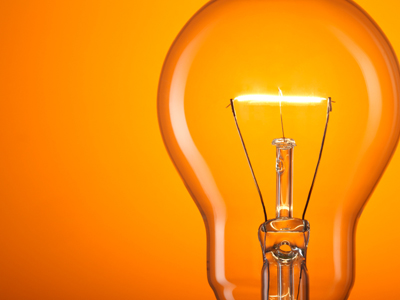In a filament lamp, most of the energy is lost as heat.

# Electricity - Current, Charge and Power

Knowing how current, charge and power are calculated is extremely helpful in GCSE Physics when considering electrical circuits. In everyday life, there is a wide choice of electrical appliances available to buy. They are all designed to transfer electrical energy into other forms of energy, but not all appliances are as efficient as one another.

Knowing how current, charge and power are related can help you decide when you are buying. It can also help you with safety in the home as you can work out the current that each one will draw when running at full power. So how is this useful? You can work out the correct size of fuse to use and also make sure that an extension lead or household circuit is not being overloaded.

Electricity is a flow of electrons through a material. Electrons carry a very small amount of electrical charge but since they are extremely tiny, there are massive numbers of them on the move in a working circuit. One coulomb equals the negative of the charge of 6.24 x 1018 electrons. Using the coulomb avoids needing to use this large number in calculations. In equations, charge is represented by q (or Q) and has the SI unit symbol C.

Electrical current is the rate of flow of this charge in a circuit. It has the symbol I in equations and is measured in amperes (usually shortened to 'amps') which has the SI unit symbol of A. The current flowing round a circuit is measured using an ammeter. One amp is equivalent to one coulomb of electricity passing a given point in a circuit in one second.

Power refers to how fast energy is transferred, so you can calculate the power consumption (in watts) of an electrical appliance by dividing the energy transferred (in joules) by the time taken to transfer that amount of energy in seconds. One of the common mistakes at GCSE is to forget to convert time into seconds. Power is directly related to the current and the potential difference, if you multiply the curent in amps by the potential difference in volts, you have the power of an appliance. In the exams, when asked about electricity you will often need to rearrange these relationships (or even combine them) to calculate the figure demanded in the question, so make sure that you revise the basic equations related to current charge and power thoroughly.

1.
If a charge flows through a resistor, which of the following happens to the resistor?
It gets colder
It gets hotter
It becomes positively charged
Nothing
A resistor makes it harder for the electrons to pass and some of their energy is transferred into heat by the resistor
2.
In a filament lamp, in what form is most of the energy lost?
Heat
Sound
Kinetic energy
The filament is a resistance wire that is designed to become so hot that it emits energy in the visible light part of the spectrum
3.
What is power?
The number of repetitions of squats a body-builder can perform
The number of cylinders in a car
The rate at which energy is absorbed
The rate at which energy is transferred
In everyday language, power can have several meanings but in physics, it always means the same thing whether it is in an electrical or a mechanical context
4.
What is the formula for power?
P = Et
P = tE
P = E2t
P = 2Et
If the units you use for energy and time are joules and seconds, the power will be in watts
5.
Calculate the power of an appliance that uses 1000 joules of energy in 5 seconds.
100 W
200 W
300 W
400 W
Power is the rate at which energy is transferred therefore you need to divide the energy by the time during which it was being transferred. 1000 divided by 5 gives you the answer of 200. Since the energy transferred is in joules and the time in seconds, the power has been calculated in watts
6.
Power, potential difference and current are correctly related by which equation?
P = I x V
P = 2I x V
P = IV
P = I2V
This is a very handy equation - make sure you learn it well and can rearrange it to work out the current or potential difference
7.
What is the voltage of an appliance if it uses 200 J per second when supplied with a current of 5 A?
10 V
20 V
30 V
40 V
Always ensure you give units in your answer. Marks can be lost for missing them out!
8.
Which equation correctly shows the relationship between energy transferred, voltage and charge?
E = V x Q
E = 2V x Q
E = VQ
E = QV
You can substitute current and power in place of voltage if it is not given in a question. You need to use this formula and combine it with the one given in question 6
9.
What is the energy transferred if the voltage is 5 V and the charge is 10 C?
50
25
50 J
25 J
Whilst you would get a mark for answering 50 you would lose a mark for not giving units with your answer. If you are struggling to calculate an answer to a numerical question, always put a number with the correct units! At least you will get a mark for using the correct units
10.
What size of fuse would be required for a 230 V, 500 W heater?
3 A
5 A
13 A
1 A
To find out the most appropriate fuse to use, calculate the current flowing through the appliance. The fuse should have a rating slightly higher than the current